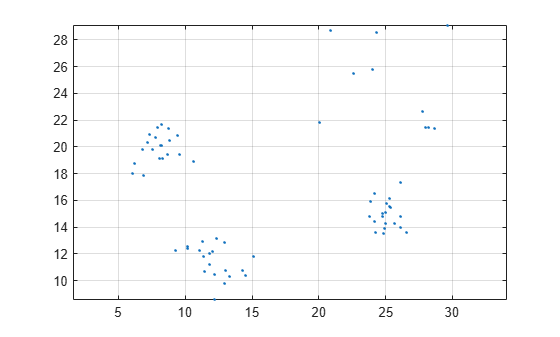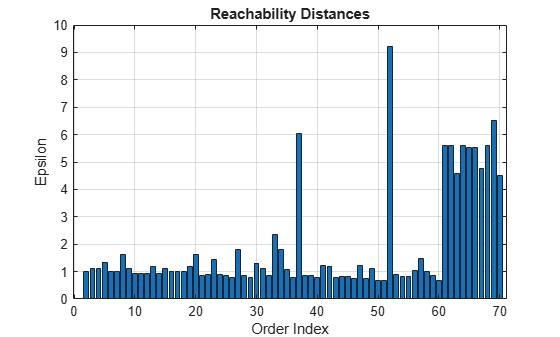# clusterDBSCAN.discoverClusters

Find cluster hierarchy in data

## Syntax

``[order,reachdist] = clusterDBSCAN.discoverClusters(X,maxepsilon,minnumpoints)``
``clusterDBSCAN.discoverClusters(X,maxepsilon,minnumpoints)``

## Description

example

````[order,reachdist] = clusterDBSCAN.discoverClusters(X,maxepsilon,minnumpoints)` returns a cluster-ordered list of points, `order`, and the reachability distances, `reachdist`, for each point in the data `X`. Specify the maximum epsilon, `maxepsilon`, and the minimum number of points, `minnumpoints`. The method implements the Ordering Points To Identify the Clustering Structure (OPTICS) algorithm. The OPTICS algorithm is useful when clusters have varying densities.```
````clusterDBSCAN.discoverClusters(X,maxepsilon,minnumpoints)` displays a bar graph representing the cluster hierarchy.```

## Examples

collapse all

Create target data with random detections in xy Cartesian coordinates. Use the `clusterDBSCAN.discoverClusters` object functions to reveal the underlying cluster hierarchy.

First, set `clusterDBSCAN.discoverClusters` parameters.

```maxEpsilon = 10; minNumPoints = 6;```

Create random target data.

```X = [randn(20,2) + [11.5,11.5]; randn(20,2) + [25,15]; randn(20,2) + [8,20]; 10*rand(10,2) + [20,20]]; plot(X(:,1),X(:,2),'.') axis equal grid```Plot the cluster hierarchy.

`clusterDBSCAN.discoverClusters(X,maxEpsilon,minNumPoints)`From a visual inspection of the plot, choose `Epsilon` as 2 and then perform the clustering using the `clusterDBSCAN` object and plot the resultant clusters.

```clusterer = clusterDBSCAN('MinNumPoints',6,'Epsilon',2, ... 'EnableDisambiguation',false); [idx,cidx] = clusterer(X); plot(clusterer,X,idx)```## Input Arguments

collapse all

Input feature data, specified as a real-valued N-by-P matrix. The N rows correspond to feature points in a P-dimensional feature space. The P columns contain the values of the features over which clustering takes place. The DBSCAN algorithm can cluster any type of data with appropriate `MinNumPoints` and `Epsilon` settings. For example, a two-column input can contain the xy Cartesian coordinates, or range and Doppler.

Data Types: `double`

Maximum epsilon size to use in the cluster hierarchy search, specified as a positive scalar. The epsilon parameter defines the clustering neighborhood around a point. Reducing `maxepsilon` results in shorter run times. Setting `maxepsilon` to `inf` identifies all possible clusters.

The OPTICS algorithm is relatively insensitive to parameter settings, but choosing larger parameters can improve results.

Example: `5.0`

Data Types: `double`

Minimum number of points used as a threshold, specified as a positive integer. The threshold sets the minimum number of points for a cluster.

The OPTICS algorithm is relatively insensitive to parameter settings, but choosing larger parameters can improve results.

Example: `10`

Data Types: `double`

## Output Arguments

collapse all

Cluster ordered list of sample indices, returned as an integer-valued 1-by-N row vector.N is the number of rows in the input data matrix `X`.

Reachability distance, returned as a positive, real-valued 1-by-N row vector. N is the number of rows in the input data matrix `X`.

Data Types: `double`

## Algorithms

The outputs of `clusterDBSCAN.discoverClusters` let you create a reachability-plot from which the hierarchical structure of the clusters can be visualized. A reachability-plot contains ordered points on the x-axis and the reachability distances on the y-axis. Use the outputs to examine the cluster structure over a broad range of parameter settings. You can use the output to help estimate appropriate epsilon clustering thresholds for the DBSCAN algorithm. Points belonging to a cluster have small reachability distances to their nearest neighbor, and clusters appear as valleys in the reachability plot. Deeper valleys correspond to denser clusters. Determine epsilon from the ordinate of the bottom of the valleys.

OPTICS assumes that dense clusters are entirely contained by less dense clusters. OPTICS processes data in the correct order by tracking the point density neighborhoods. This process is performed by ordering data points by the shortest reachability distances, guaranteeing that clusters with higher density are identified first.

## Extended Capabilities